# Representations are adjoint to endomorphisms

## Abstract

The functor that takes a ring to its category of modules has an adjoint if one remembers the forgetful functor to abelian groups: the endomorphism ring of linear natural transformations. This uses the self-enrichment of the category of abelian groups. If one considers enrichments into symmetric sequences or even bisymmetric sequences, one can produce an endomorphism operad or an endomorphism properad. In this note, we show that more generally, given a categoryenriched in a monoidal category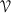, the functor that associates to a monoid inits category of representations in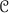is adjoint to the functor that computes the endomorphism monoid of any functor with domain. After describing the first results of the theory we give several examples of applications.

This is a preview of subscription content, access via your institution.

## Change history

• ### 06 March 2020

The first equation under section “Remark 3” was processed and published incorrectly. The correct equation should read as follows:

## References

1. 1.

Drummond-Cole, G.C., Hirsh, J., Lejay, D.: Endomorphism operads of functors. ArXiv e-prints arXiv:1906.09006 [math.CT] (2019)

2. 2.

Yoneda, N.: On Ext and exact sequences. J. Fac. Sci. (Imperial University of Tokyo) 8, 507–576 (1960)

3. 3.

Yau, D.: Colored operads, Graduate Studies in Mathematics, vol. 170. American Mathematical Society, Providence (2016)

4. 4.

Grignou, B.L., Lejay, D.: Homotopy theory of linear cogebras. ArXiv e-prints arXiv:1803.01376 [math.AT] (2018)

5. 5.

Janelidze, G., Kelly, G.M.: A note on actions of a monoidal category. Theory Appl. Categ. 9, 61–91 (2001)

6. 6.

Joyal, A.: Foncteurs analytiques et espèces de structures. In: Combinatoire énumérative, pp. 126–159. Springer, Berlin (1986). https://doi.org/10.1007/BFb0072514

7. 7.

Grignou, B.L., Lejay, D.: Operads without cogebras, ArXiv e-prints arXiv:1902.02551 [math.AT] (2019)

8. 8.

Kelly, G.M.: Basic Concepts of Enriched Category Theory, London Mathematical Society Lecture Note Series, vol. 64. Cambridge University Press, Cambridge (1982)

9. 9.

Ross, S.: Quantum Groups: A Path to Current Algebra, Australian Mathematical Society Lecture Series, vol. 19. Cambridge University Press, Cambridge (2007)

10. 10.

Arone, G., Ching, M.: Operads and chain rules for the calculus of functors, vol. 338 of Astérisque. Société Mathématique de France (2011)

11. 11.

Vallette, B.: Koszul duality for PROPs. Comptes Rendus Mathematique 338(12), 909–914 (2004)

12. 12.

Gan, W.L.: Koszul duality for dioperads. Math. Res. Lett. 10(1), 109–124 (2003)

13. 13.

Yau, D., Johnson, M.: A Foundation for PROPs, Algebras, and Modules. American Mathematical Society, Providence (2015)

14. 14.

Joyal, A., Ross, S.: An Introduction to Tannaka duality and quantum groups. In: Lecture Notes in Mathematics, pp. 413–492. Springer, Berlin (1991)

15. 15.

Anel, M., Joyal, A.: Sweedler Theory for (co)algebras and the bar-cobar constructions. ArXiv e-prints arXiv:1309.6952 [math.CT] (2013)

16. 16.

Montgomery, S.: Hopf Algebras and Their Actions on Rings. American Mathematical Society, Providence (1993)

## Acknowledgements

The authors would like to thank Rune Haugseng, Theo Johnson-Freyd, Johan Leray, Emily Riehl, and Claudia Scheimbauer for useful discussions, as well as Greg Arone and Birgit Richter for pointing us to relevant literature.

## Author information

Authors

### Corresponding author

Correspondence to Damien Lejay.

### Publisher's Note

Springer Nature remains neutral with regard to jurisdictional claims in published maps and institutional affiliations.

Gabriel C. Drummond-Cole and Damien Lejay were supported by IBS-R003-D1.

The original version of this article was revised to correct the table under “Introduction” section and an equation under “Remark 3” section.

Communicated by Jiri Rosicky.

## Appendices

### Terminology of enriched categories

We let the reader turn to Kelly  for a detailed exposition on categories enriched in a monoidal category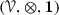. In order to not be bothered by size issues, we fix once and for all three infinite inaccessible cardinals L < XL < XXL and use the dictionary

\begin{aligned} \text {small} :=\text {L-small}; \quad \text {large} :=\text {XL-small}; \quad \text {very large} :=\text {XXL-small}. \end{aligned}

We now assume thatis large (has large sets of objects and morphisms) and has all small limits and colimits. In what follows we consider a large-enriched category

and assume thatis large.

### Enlargement of the universe

For convenience (when computing over large diagrams), we shall enlarge: we choose a very large monoidal category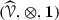with a full monoidal embedding

The enlarged universe can be chosen to be locally large, have all large limits and colimits and the embedding can be assumed to commute with small limits and colimits. This is discussed for example by Kelly [8, §2.6] (albeit in the closed symmetric setting).

The-categorycan now without effort be seen as a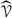-category

### Definition 3

(Closed monoidal category) One says thatis closed when the functor $$Y \mapsto Y \otimes X$$ has a right adjoint $$Z \mapsto X^Z$$ for each object X in.

### Definition 4

(Tensored) One says thatis tensored overwheneveris closed and for every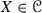and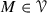, the functor

\begin{aligned} Y \longmapsto {[X,Y]}^M \end{aligned}

is-representable by an object denoted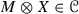. In that case, sinceis closed the induced functor

is naturally endowed with a monoidal structure.

### Definition 5

(Faithfully tensored) We shall say thatis faithfully tensored overif it is tensored and the functor

is faithful.

### Definition 6

(Accessibly tensored) We shall say thatis accessibly tensored overif it is tensored, bothandare accessible and for every, the functor

is accessible.

### Definition 7

(Accessibly enriched) Whenandare both accessible, we shall say thatis accessibly enriched if there exists a small cardinal $$\kappa$$ such that for every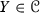, the functor

commutes with $$\kappa$$-cofiltered limits.

### Remark 8

One can check that ifis accessibly tensored, it is then accessibly enriched.

### Examples of contexts of application

In this appendix, we give several application contexts for the adjunction

In each context, the terminology is specific, both for monoids and for their categories of representations.

### Using a closed symmetric monoidal category

In the next examples, we fix a presentable closed symmetric monoidal categoryand denote its internal hom by $$\langle - ,- \rangle$$. We then consider several enrichments for.

Potential examples of such closed symmetric monoidal categories include the category of sets, vector spaces or coassociative cogebras (more generally cogebras over Hopf operads). It also includes the categories of sheaves valued in those categories.

#### Self enrichment

This one is the most obvious, since the monoidal structure ofis closed, it is self-enriched via

\begin{aligned}{}[X, Y] :=\langle X, Y \rangle . \end{aligned}

In this context, the general idea of the adjunction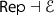was well-known to people doing reconstruction theorems à la Tannaka. It appears for example in Street’s Quantum groups: a path to current algebra [9, Ch. 16].

Let us denote bythe category of symmetric sequences: sequences of objects M(n) ofendowed with right $$\mathbf{S }_{n}$$-actions for every natural n. The categoryis accessibly tensored over the category of symmetric sequences via the formula

\begin{aligned} M \mathbin {\triangleleft }X :=\coprod _{n \in \mathbf{N} } M(n) \otimes _{\mathbf{S }_{n}} X^{\otimes n}. \end{aligned}

This induces a monoidal structure on symmetric sequences

\begin{aligned} M \mathbin {\triangleleft }N :=\coprod _{n \in \mathbf{N} } M(n) \otimes _{\mathbf{S }_{n}} N^{\circledast n}. \end{aligned}

Where $$\circledast$$ denotes the convolution of symmetric sequences. The associated enrichment is given by

\begin{aligned}{}[X, Y](n) :=\langle X^{\otimes n}, Y \rangle . \end{aligned}

Monoids in symmetric sequences are called operadsGiven an operad P, its category of representations is called the category of P-algebras. One thus gets an adjunction

#### The other (cogebraic) operadic enrichment

This time we let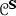be the category of symmetric sequences with left actions of the symmetric groups. It admits a monoidal structure given by

\begin{aligned} M \mathbin {\triangleright }N :=\coprod _{n \in \mathbf{N} } M^{\circledast n} \otimes _{\mathbf{S }_{n}} N(n) \end{aligned}

and the associated enrichment is

\begin{aligned}{}[X, Y](n) :=\langle X, Y^{\otimes n} \rangle . \end{aligned}

Since left and right actions of symmetric groups are equivalent, one has an equivalence of categories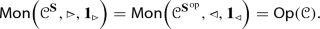In this case, the category of representations of an operad P is its category of cogebras. Conversely, the functor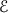associates to a functor F, seen as an object of the functor category, its coendomorphism operad.

In general, the category of P-cogebras may not be presentable, although (for example) it is presentable if the ground category is dg-vector spaces . Thus, one has the adjunction

This example arises naturally in applications and appeared, for example, in unpublished work by May, who considered it well-known.

In one application, the singular chains functor from topological spaces to chain complexes factors through the category of $${\mathsf {E}}_\infty$$-cogebras in chain complexes.

The following stable improvement of this example was pointed out to us by Arone: the coendomorphism operad of the suspension functor from pointed spaces to spectra can be shown to be weakly equivalent to the commutative operad .

#### Propic enrichments

Going further, one can enrichin the category of bisymmetric sequencesusing

\begin{aligned}{}[X, Y](p, q) :=\langle X^{\otimes p}, Y^{\otimes q}\rangle . \end{aligned}

There are several monoidal structures on bisymmetric sequences compatible with these enrichment objects, depending on the classes of graphs involved in the definition of the monoidal structure. One can allow connected graphs, in which case the monoids are properads [11, 2.1], or allow only simply connected graphs, in which case the monoids are dioperads [12, 4.2]. Similar but more exotic examples are also possible .

### Examples with exogenous enrichments

#### Representations of topological monoids

The following example is taken from the duality between topological groups and their categories of representations due to Tannaka . The category of finite dimensional vector spaces is canonically enriched in topological spaces. Since this category is small, one gets an adjunction

whereassociates to any functor $$F : {\mathcal {D}}\rightarrow {\mathsf {Vect}}_{\text {fd}}$$ its topological monoid of endomorphisms.

#### Bigebras

Let $${\varvec{K}}$$ be a field. The category of associative $${\varvec{K}}$$-algebras is naturally cotensored over $${\varvec{K}}$$-cogebras: given a cogebra V and an algebra $${\varLambda }$$, convolution gives $$\text {Hom}_{{\varvec{K}}}(V, {\varLambda })$$ a structure of associative algebra. This cotensorization comes with an enrichment and a tensorization .

Monoid objects in cogebras are bigebras. Given a bigebra H, it is an exercise to verify that the category of representations $$H\text {-}{\mathsf {rep}}$$ is naturally isomorphic to the category of H-module algebras studied by Hopf theorists [16, 4.1.1] equipped with the functor to algebras forgetting the H-module structure. We thus obtain an adjunction

where for an accessible functor $$F :{\mathcal {D}}\rightarrow {\mathsf {Alg}}$$, the endomorphism bigebrais universal among bigebras acting compatibly on the objects of $${\mathcal {D}}$$.

## Rights and permissions

Reprints and Permissions